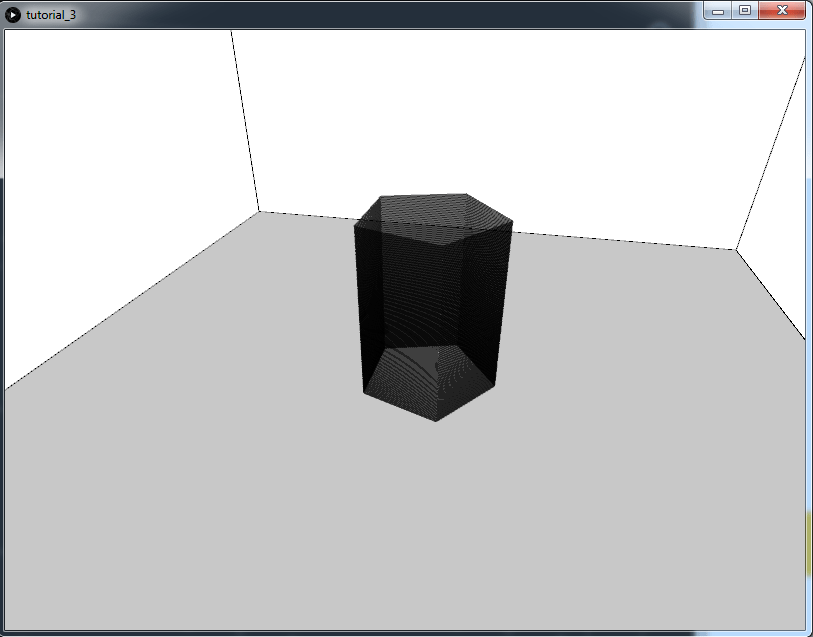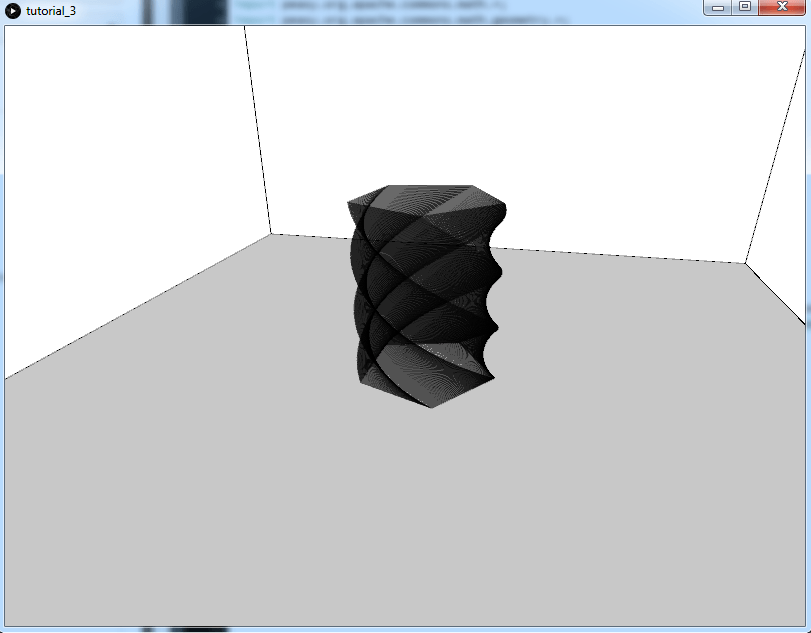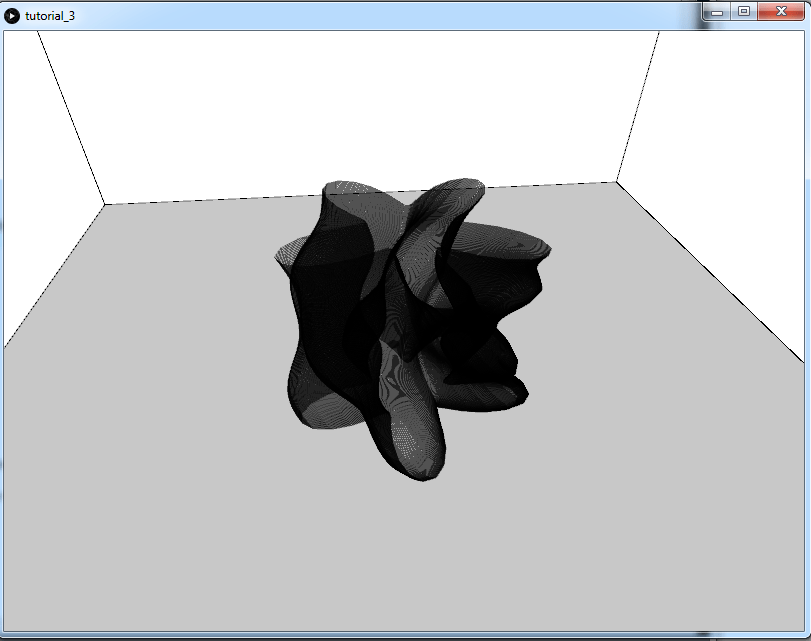# Vase generator with Processing for 3D printing – (Part 1)

In the previous tutorial we have implemented a possible structure of classes for generating G-code with Processing, now we will apply it for something more interesting than our usual cube 🙂 : a vase generator.

If we did things properly before, today we will only have to focus on the `Creator` class. Let’s start.

## Creating a Vase Generator

First we create a new inherited class from `Creator`: let’s call it Vase. We will set in the constructor its position, while the other instances will have default values. To modify them we will use methods that return the same `Vase` object so that we can use method chaining.

```class Creator {
ArrayList<Path> paths = new ArrayList<Path>();

Printer printer;
Settings settings;

Creator(Printer t_printer, Settings t_settings) {
printer = t_printer;
settings = t_settings;
}
}

class Vase extends Creator {
float center_x;
float center_y;

float len = 10;
float wid = 10;
float hei = 10;

int sides = 4;

Vase(Printer t_printer, Settings t_settings, float c_x, float c_y) {
super(t_printer, t_settings);
center_x = c_x;
center_y = c_y;
}

Vase setCenter(float x, float y) {
center_x = constrain(x, 0, printer.width_table);
center_y = constrain(y, 0, printer.length_table);
return this;
}

Vase setLength(float l) {
len = constrain(l, settings.path_width, max(printer.width_table, printer.length_table));
return this;
}

Vase setWidth(float w) {
wid = constrain(w, settings.path_width, max(printer.width_table, printer.length_table));
return this;
}

Vase setWidthAndLength(float wl) {
wid = constrain(wl, settings.path_width, max(printer.width_table, printer.length_table));
len = constrain(wl, settings.path_width, max(printer.width_table, printer.length_table));
return this;
}

Vase setHeight(float h) {
hei = constrain(h, settings.layer_height, printer.height_printer);
return this;
}

Vase setSides(int n) {
sides = n;
return this;
}
}```

Now we create a `generate()` method for our class, similar to the one that we have seen in the previous tutorial for the cube:

```class Vase extends Creator {

//...

Vase(Printer t_printer, Settings t_settings, float c_x, float c_y) {
super(t_printer, t_settings);
center_x = c_x;
center_y = c_y;
}

void generate() {

paths = new ArrayList<Path>();

float tot_layers = hei / settings.layer_height;
float z = 0;

float angle_increment = TWO_PI / (float)sides;

for (int layer = 0; layer<tot_layers; layer++) {

z += settings.layer_height;
paths.add(new Path());

for (float angle = 0; angle<=TWO_PI; angle+=angle_increment) {

float x = center_x + cos(angle) * len;
float y = center_y + sin(angle) * wid;

PVector next_point = new PVector(x, y, z);

paths.get(paths.size()-1).addPoint(next_point);
}
}
}

//...

}```

Let’s test it:

```void setup() {
size(800, 600, P3D);

cam = new PeasyCam(this, 100);

_printer = new Printer();
_settings = new Settings();

Vase vase1 = new Vase(_printer, _settings, _printer.x_center_table, _printer.y_center_table);
vase1.setSides(5).setWidthAndLength(20).setHeight(50).generate();

_processor = new Processor();
_processor.addObject(vase1);
_processor.sortPaths();

_drawer = new Drawer(_processor, _printer);

_gcodeGenerator = new GcodeGenerator(_printer, _settings, _processor);
_gcodeGenerator.generate().export();
}

void draw() {
background(255);

_drawer.displayAll(color(0,150));
}```Now we can start to make things more interesting. How about applying a rotation to our cube?

```class Vase extends Creator {
float center_x;
float center_y;

float len = 10;
float wid = 10;
float hei = 10;

int sides = 4;

float rotation_increment = 0;

Vase(Printer t_printer, Settings t_settings, float c_x, float c_y) {
super(t_printer, t_settings);
center_x = c_x;
center_y = c_y;
}

void generate() {

paths = new ArrayList<Path>();

float tot_layers = hei / settings.layer_height;
float z = 0;

float angle_increment = TWO_PI / (float)sides;

float rotation = 0;

for (int layer = 0; layer<tot_layers; layer++) {

z += settings.layer_height;
rotation += rotation_increment;
paths.add(new Path());

for (float angle = 0; angle<=TWO_PI; angle+=angle_increment) {

float x = center_x + cos(angle + rotation) * len;
float y = center_y + sin(angle + rotation) * wid;

PVector next_point = new PVector(x, y, z);

paths.get(paths.size()-1).addPoint(next_point);
}
}
}

//...

Vase setRotation(float r) {
rotation_increment = r;
return this;
}

}
```

```void setup() {
size(800, 600, P3D);

//...

Vase vase1 = new Vase(_printer, _settings, _printer.x_center_table, _printer.y_center_table);
vase1.setSides(5).setWidthAndLength(20).setHeight(50).setRotation(PI/200).generate();

//...
}```Now we will add some oscillations on the three axes.

```class Vase extends Creator {

//...

float amount_oscillation_XY = 0;
float increment_oscillation_XY = 0;

float amount_oscillation_Z = 0;
float increment_oscillation_Z = 0;

//...

void generate() {

paths = new ArrayList<Path>();

float tot_layers = hei / settings.layer_height;
float z = 0;

float angle_increment = TWO_PI / (float)sides;

float rotation = 0;

float oscillation_Z = 0;

for (int layer = 0; layer<tot_layers; layer++) {

z += settings.layer_height;
rotation += increment_rotation;
oscillation_Z += increment_oscillation_Z;

Path new_path = new Path();

float oscillation_XY = 0;

for (float angle = 0; angle<=TWO_PI; angle+=angle_increment) {

oscillation_XY+=increment_oscillation_XY;

float x = center_x + cos(angle + rotation) * (len + sin(oscillation_XY) * amount_oscillation_XY + sin(oscillation_Z) * amount_oscillation_Z);
float y = center_y + sin(angle + rotation) * (wid + sin(oscillation_XY) * amount_oscillation_XY + sin(oscillation_Z) * amount_oscillation_Z);

PVector next_point = new PVector(x, y, z);

new_path.addPoint(next_point);
}

new_path.makeClosed();
paths.add(new_path);
}
}

//...

Vase setOscillationXYAmount(float w_l_xy) {
amount_oscillation_XY = w_l_xy;
return this;
}

Vase setOscillationXY(float w_xy) {
increment_oscillation_XY = w_xy;
return this;
}

Vase setOscillationZAmount(float w_l_z) {
amount_oscillation_Z = w_l_z;
return this;
}

Vase setOscillationZ(float w_z) {
increment_oscillation_Z = w_z;
return this;
}
}```

```void setup() {

//...

Vase vase1 = new Vase(_printer, _settings, _printer.x_center_table, _printer.y_center_table);
vase1.setSides(60)
.setWidthAndLength(20)
.setHeight(50)
.setRotation(0.005)
.setOscillationXY(0.5)
.setOscillationXYAmount(10)
.setOscillationZ(0.05)
.setOscillationZAmount(2);
vase1.generate();

//...

}```With the same technique we can apply many more edgy transformations: how about a second layer of oscillations? Or add some Perlin noise on the three axis? Can you skew the hole shape as a spiral? Try it yourself! Remember that you can create more than one object, you just need to pass all of them at the end to the `Processor`. Maybe you can think of a way (like a new class) of processing multiple objects at the same time, dispose them in a grid or other pattern. Experiment and show us what you came up with!

What we have written so far is good and is working, however it is a little bit annoying to set the parameters and restarting the program every time; it would be much better if we could visualize the changes on the fly and then export the G-code when we are happy with the results. That’s what we are going to do in the next tutorial, by adding a GUI to our vase.

Stay tuned!## 2 comments

1.Alan Smith Krusemark says:

The function makeClosed() does not exist. Do you have this function available? It’s not detailed in your tutorial.